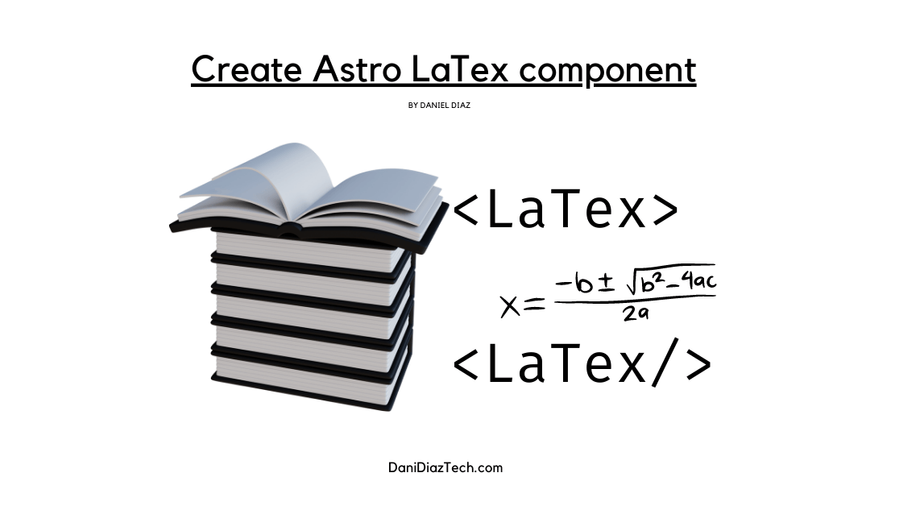# How to create an Astro LaTeX component/* In this tutorial, you're going to learn how to create an Astro component to display LaTeX in your markdown or MDX files. */

I built a custom LaTeX Astro component, to be able to display math formulas on my website. Especially for my posts written in MDX.

In this quick guide, I’ll show you I did it

The first thing you need is a way to parse LaTeX strings into HTML code. You don’t need to write a parser from scratch.

The KaTeX library does a fantastic job, and because it provides server-side rendering, you don’t need to worry about a decrease in performance on your site — Astro builds your site in the server.

If you create content with Astro, you can use MDX files to use components you’ve already created.

Run the following at the root of your project, to install both packages.

npm i katex
npx astro add mdx

You should have something like the following in the package.json file.

{
// ...
"dependencies": {
// More dependencies
"katex": "^0.16.4"
}
}

And the MDX integration in your astro.config.mjs file.

import { defineConfig } from 'astro/config';
import mdx from '@astrojs/mdx';

export default defineConfig({
// More configs
integrations: [mdx()],
});

## Creating The LaTeX Component permalink

This Astro component is very simple. We take the formula as a prop, transform it to HTML with KaTeX, and render it a <span> tag, using the set:html directive.

Note

The set:html directive injects HTML code into an element, in this case a span tag.

Create an Astro file like Latex.astro (I prefer this name, rather than the grammatically correct one) in your components folder (src/components), and paste the following code.

---
import katex from 'katex';

const { formula = '' } = Astro.props;
const html = katex.renderToString(formula, {throwOnError: false});
---

<div class="text-center">
<span set:html={html} class="" />
</div>

rel="stylesheet"
href="https://cdn.jsdelivr.net/npm/katex@0.15.1/dist/katex.css"
integrity="sha384-WsHMgfkABRyG494OmuiNmkAOk8nhO1qE+Y6wns6v+EoNoTNxrWxYpl5ZYWFOLPCM"
crossorigin="anonymous"
/>

The link to the stylesheet is necessary to render the LaTeX string correctly. We, set the throwOnError option to false, so if we type an incorrect formula our site won’t break.

## Using the LaTeX Component in MDX files permalink

Now, create an MDX file (the .mdx extension is required) to test this component. You need to import it with a relative path — relative to the folder you’re writing the MDX file from.

Note

You can also use this component in regular Astro pages, as you would do with any other component!

---
title: Testing my LaTeX component
{/* Other frontmatter content */}
---
import Latex from '../components/Latex.astro'.
<Latex formula='E=mc^2'/>

Here is what the formula should look like.

$E=mc^2$

You can write any complex LaTeX formula, it’ll be rendered correctly by katex. But if the string of the formula gets pretty large, you might want to use constants to store them.

For example, here is matrix represented in LaTeX.

import Latex from '../components/Latex.astro'.
export const f1 = '\\begin{bmatrix}a & b \\\\ c & d\\end{bmatrix}';
<Latex formula={f1}/>
$\begin{bmatrix}a & b \\ c & d\end{bmatrix}$

When writing formulas, note that you must escape the backslash (making a double backslash).

import Latex from '../components/Latex.astro'.
export const f2 = '\\overbrace{a+b+c}^{\\text{a sum of three numbers}}';
<Latex formula={f2}/>
$\overbrace{a+b+c}^{\text{a sum of three numbers}}$

You can read more about supported LaTeX elements in the KaTeX docs.